[HomePage] Prof Morris Kline

Chapter 4: The Deductive Approach to Mathematics.

The great science [mathematics] occupies itself at least as much with the power of imagination as with the power of logical conclusion.

Johann Friedrich Herbart

One of the major criticisms of the traditional curriculum is that students learn to do mathematics by rote, by memorizing procedures and proofs. It is the contention of the advocates of the modern mathematics curriculum that when the subject is taught logically, when the reasoning behind steps is revealed, students will no longer have to rely upon rote learning. They will understand the mathematics. The logical approach is, in other words, also the pedagogical approach and the panacea for the difficu1ties students have had in learning mathematics.

Just what does the logical approach mean? Basica1ly it is the one commonly used in the tradidina1 curriculum to teach high school geometry. That is, one starts with definitions and axioms and proves conclusions, called theorems, deductively. Though this approach has been used in geometry, it has not been used in the teaching of arithmetic, algebra, and trigonometry. Hence, so far as this feature of the new curriculum is concerned, the major change is in these latter subjects. Let us see what the deductive approach to arithmetic and algebra entails.
[* The reader who is familiar with the deductive approach may want to skip the examples given below]

The examples we shall examine are taken from typical modern mathematics texts.

The approach to arithmetic usually presupposes that we know what the numbers 0, 1 ,2, . . ., called the counting numbers, are. It then introduces axioms. We know that 4 added to 3 yields the same number as 3 added to 4, or 3 + 4 = 4 + 3. This axiom is stated in symbolic language as
a + b = b + a and is called the commutative axiom. That is, we may commute or interchange the order in which we add two numbers.

If we had to ca1cu1ate 3 + 4 + 5 we cou1d ca1culate 3 + 4 and then add 5 to the result or we could calcu1ate 4 + 5 and add this result to 3. That is, we cou1d first associate the 3 and 4 and then add 5 or we cou1d first associate the 4 and 5 and add the resu1t, 9, to the 3. Thus we have the associative axiom of addition. In symbolic language it states that
(a + b) + c = a + (b + c). The commutative and associative axioms apply to multiplication aIso. That is, a x b = b x a and (a x b) x c = a x (b x c).

Let us consider next 3 x (4 + 5). This is 3 x 9 or 27. It is also 3 x 4 + 3 x 5. That is, a x (b + c) = a x b + a x c. This fact is another axiom and it is called the distributive axiom. That is, we may distribute the
mu1tiplication over the
b and the c instead of applying it to b + c.

There are other axioms. For example, the sum and product of two counting numbers is a unique counting

~ The reader who is familiar with the deductive approach may want to skip the examples in the next few pages.

number. There is a unique number
0, such that a + 0 =a for each counting number a, and there is a unique
number
1 such that a x 1 = a for each counting number a.

These axioms are used to justify steps in arithmetic. Consider the addition of 38 and 3. This is performed as follows.

By the definition of 38

38 + 3 = (30 + 8) + 3.

The associative axiom tells us that

(30+8) + 3 = 30 + (8+3).

Now, by the definition of
3,

30 + (8+3) = 30 + (8 + [2 + 1])

and by the associative axiom for addition

30 + (8 + [2+1] ) = 30 + ([8 + 2] + 1).

8 and 2 yields

30 + ([8 + 2] + 1) = 30 + (10 + 1).

By the associative axiom

30 + (10 + 1) = (30 + 10) + 1,

and - since
30 + 10 = 40 and 40 + 1 = 41, we have

finally deduced that

38+3=41.

To see how the distributive axiom is used in arithmetic let us consider
7 x 1 3. By the definition of 13

7 x 13=7 x (10 + 3).

Now, the distributive axiom tells us that Since
7 x 10 = 70 and
7 x 3 = 21, we have deduced that -

7 x 13 = 91.

Presumably these steps, which employ the distributive axiom, make clearer how the 91 is arrived at than by mu1tiplying in the usual fashion wherein one says that 7 x 3 = 2 1, writes down the 1, carries the 2 over to the tens, column and adds it to the result obtained from multiplying 7 and 1.

It is true that our method of writing numbers such as 13 employs what is called positional notation. That is, the 1 in 13 stands for 10 and this must be taken into account in any method of teaching mu1tiplication. Whether citing the distributive axiom clarifies the operation is the issue. Let us suppose that it does and see what it leads to. Consider the problem of 17 x 13. To follow the. above pattern we must do as follows:

17 x 13 = (10 + 7) x (10 + 3).

Now 10 + 7 must be regarded as a single number and by the distributive axiom

(10 + 7) x (10 + 3) = [10 + 7] x 10 + [10 + 7] x 3.

By the commutative axiom of multiplication

[10 + 7] x 10 + [10 + 7] x 3=l0 x [10 +7 ] + 3 x [10 + 7],

and again by the distributive axiom

l0 x [10+7]+3 x [10 + 7]

= (10 x 10 + 10 x 7) + (3 x 10 + 3 x 7).

Ca1cu1ation of the quantities in the parentheses and addition of the two results yield
221. We can readily envision the steps that would have to be undertaken to multiply, say, 172 by 135.

At some stage in the development of arithmetic and algebra, usually between the seventh and the ninth grades, students are asked to learn negative numbers.To motivate the introduction of negative numbers students are asked first, what number x satisfies the equation 17 + x = 21 Here the answer is clearly 4. Now comes the crucial question. What number x satisfies the equation 21 + x = 17?

To answer this question, one writes 21 as 17 + 4 so that
(17 + 4) + x =17.

By the associative axiom

17 + (4 + x) = 17.

But by the definition of 0

17 + 0 = 17,

so that, since the zero is unique,

4+x=0.

We see that if there were a number x such that x + 4 = 0 then we would be able to solve the original equation. We are therefore motivated to introduce the number -4 with the understanding that 4 + (-4) = 0.

The counting numbers (except 0) are now called the positive integers and the new numbers are called the negative integers. To operate with negative integers the axioms or basic properties that apply to the counting nusnbers are now assumed to hold for the combination of the old counting numbers and the new negative numbers. Thus, since the cominutative, and associative axioms of addition hold for the counting numbers, they hold for the positive and negative integers.For example, to add -2 and -5 we note first that by the definition of -2 and -5,
(-2 + 2) +(-5 + 5) = 0 + 0 = 0.

But by the commutative and associative axioms

(-2 + 2) + (-5 + 5) = 2 + 5 + [(-2) + (-5)1, -

so that

0 - 7 + [(-2) + (-5)].

Hence
-2 + (-5) must be -7 because when it is added to 7 it gives 0.

Having Iearned how to add negative numbers and posi-tive and negative numbers students are told that sub-, tracting a number means adding its inverse. 4 is the inverse of —4 and conversely. Hence

17 - 13 = 17 + (-13) = 4
6 - 8 = 6 + (-8) = -2
-5 - (-11) = -5 + 11 = 6.

Before establishing additiona1 properties of negative nuxnbers, let us prove that a x 0 = 0 for every integer a. Since by the definition of 0,
a + 0 = a, then

a x (a + 0) = a x a.

However, by the distributive axiom

a x (a+0) = a x a + a x 0.

Hence

a x a + a x 0 = a x a.

Therefore

a x 0 = 0,

because
0 is that number which when added to any number gives b.Now students are presumably prepared to appreciate the proof that a negative integer times a positive integer is negative. That is, -3 x 4 = -12. We start with

(1)............................a x [b + (-b)]=a x 0 = 0

because
b + (-b) = 0. However, by the distributive axiom

(2)...............................a x [b + (-b)] = a x b + a x (-b).

Hence the right sides of
(1) and (2) are equa1, so that

a x b + a x (-b) = 0.

Then
a x (-b) must be -(a x b) because a x (-b) added to a x b gives 0 and this is true only for the additive inverse of a x b, namely, -(a x b).
Finally, if in place of

-a x [b + (-b)]

and carry through the same steps with —a replacing a, we can prove that

-a x -b = a X b.

To introduce
signed (positive and negative) numbers another method is often used. One starts with the num-bers, 1,2,3,4, . . .; these are the counting numbers except for 0, and are called the natural numbers. Then an integer is defined as the equivalence class of ordered pairs of natural numbers. What this means is the following. An ordered pair of natural numbers is the pair (7,5). This, intuitively, means 7 - 5. However, (6,4), (4,2), (8,6), and millions of other pairs represent the same integer. Two such ordered pairs (a,b) and (c,d) are called equiva1ent if a + d = b + c. Hence the integer 2 is the class of all ordered pairs eqilivalent to, say (7,5). The merit of this definition is that one can, using on1y the natura1 numbers, introduce the ordered pair (5,7), which intuitively represents 5 - 7, or -2. Again (5,7), (4,6), (6,8), and so on are the same nega-tive integer, -2. The integer which we usua1ly denote by 0 is the class of ordered pairs (5,5), (6,6), (7,7), and so on. In the previous method we had to create the negative numbers. In the present method we construct the negative numbers.

Under this approach the operations with positive and negative integers are defined in terms of the ordered pairs. Thus, the sum of (7,5) and (6,3) is (13,8). Intuitively we have 2 + 3 = 5, but the logica1 development calls for the former. More generally
(a,b) + (c,d) = (a + c, b + d). We see that for the negative integers the definition of sum works in the same way. Thus (5,7) + (3,6) = (8,13) or, intuitively, -2 + -3 = -5. The ordered pair definition can be used to introduce stubtraction, multiplication and division of integers, and miraculously one obtains the usual laws for handling positive and negative integers.

To introduce fractions an approach similar to that used to introduce negative numbers is employed. The student is asked to find the value of x for which 3x = 6. Clearly x = (1/3) x 6 or 6/3. He is then asked to find the x for which 3x = 7. No integer will satisfy this equation. Hence we create new numbers, the fractions. In particular we create for x the number 7/3, which means (1 /3) x 7, and we agree that 3 x 1/3 = 1. Having introduced the fractions we agree further that the commutative, associative and distributive axioms are to apply to them. We can then prove that the usual operations for the addition, subtraction, multiplication and division of fractions apply.

In the case of fractions, too, some texts introduce them as ordered pairs of signed integers. Thus (3,5) is a fraction which intuitively means 3/5. The operations are defined in terms of the ordered pairs and then one can prove that addition and multiplication are commu-tative, associative and distiibutive.

The deductive approach encounters a serious obstacle, at least on the elementary level, in treating. irrational numbers. The reader may recall that numbers such as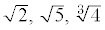and the like are also members of the number system. The full logical treatment of. these nfimbers is much too difficult to be understood by tyros, and even if the students could assimilate the material the time re-quired to teach it would be inordinate. Hence the texts usually comproxnise. They "motivate" the introduction of such numbers by first showing that to solve

x2=4

we take the square root of both sides and obtain
x = ±2.

Similarly to solve

x2 = 2

one takes the square root of both sides and obtains
x = ± \/2.

Thus one is led to introduce the irrational numbers. One can indeed prove, and many texts do, that 2 is not a rationa1 number; that is, it is not equai to a quotient of two integers. So it is clear that objects such as 2 are new kinds of numbers. But the "motivation" used above does not suffice to introduce all irrationa1 numbers,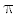in particular. It also does not serve as a logical basis on which to build the properties of irrational numbers. Consequently these properties have to be postulated. For
example, it is true, if a and b are greater than zero, that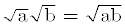But since it cannot be proved on an elementary level the texts just assert it and give it a name. It is called the
product property of square roots. Likewise the fact that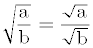cannot be proved, and so is merely asserted and labeled the quotient property for square roots.

Other difficulties arise in the introduction of complex numbers, that is, numbers of the form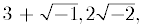etc. The new curriculuni, in this case like the traditional one, usually invents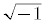as the solution of
x
2 = -1 and then forms the complex numbers. Alternatively it introduces complex numbers as ordered pairs of real numbers. It then defines the arithmetic operations with complex numbers and proves that the associative, commutative and distributive properties apply to these operations.

The logical foundation of arithmetic now serves to build algebra. Algebra is distinguished from arithnietic in that it deals with expressions involving letters such as 3x2 + 5x + 2 and operates with such expressions. Since the letters stand for numbers and all numbers obey the same laws, the letters obey these laws. Let us consider one algebraic proof. We shall prove that if a x b = 0, then either a is 0 or b is 0 or both are zero.

If a = 0 the theorem is proved. If a does not equal zero then a property of the number system tells us that there is an inverse, 1/a. Then, since
a x b = 0 and any number mu1tiplied by 0 yields 0,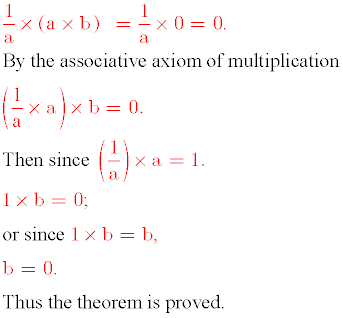Some of the textbook authors wish to cut short the logica1 development of the number system or to "innovate". The authors of one widely used text, in introducing negative numbers, list many of the usual properties:

the sum of any two integers is an integer, the commutative, associative, and distributive properties and such peculiar ones as the property of the opposite of a sum:

-(a + b) = -a + (-b).

They give examples of the uses of these properties which students are asked to imitate. Some thirty or forty properties are finally listed and the students are expected to learn and apply them. The authors do not say whether these properties are axioms nor do they prove the assertions. The net effect is confusion about what is proved from axioms and what are rules. In effect the authors are handing down rules despite their claims to be teaching deductive mathematics.

Our examples of the way in which deduction is applied to arithmetic and algebra have been very simple. One can readily imagine how lengthy and involved the proofs are when doing more complicated arithmetic and algebra.

The deductive approach to Euclidean geometry is essentially the one most adults learned in high school. Hence it is not necessary to illustrate it here. However, we shall have more to say about proof in geometry in the next chapter.

Since the major innovation of the new mathematics is the deductive approach to traditional subject matter, let us try to determine what pedagogical merit it may have. In particular, does it impart understanding of mathematics?

A number of considerations oblige us to answer this question negatively. First, let us examine how mathematics itself developed and see whether this history furnishes any evidence favoring one conclusion or another. After all, mathematics was created by human beings who certainly understood the subject. How did the masters Euclid, Archimedes, Newton, Euler, and Gauss come to understand mathematics?

Mathematics in a significant sense begins with the contributions of the Egyptians and Babylonians during the period of roughly 3000 to 300 B.C. These two peoples created the rudiments of arithinetic, algebra, and geometry. In arithmetic they worked with the positive whole numbers and fractions. Negative numbers were unknown to them and even the zero was not introduced despite the fact that Babylonians used positional notation in base sixty to write Iarge numbers. That is, in their syinbolism a number such as 125 meant 1.(60)2 + 2.60 + 5, just as in our base ten 125 means 1.102 + 2.10 + 5. Such a system of writing quantity ahiiost cries out for a zero, because to write 105 one needs the 0 to indicate that the 1 is not in the "tens" position but in the "hundreds". Yet the Babylonians did not create the zero. Their numbers were ambiguous and one had to judge from the context as to what was intended.

In geometry all that the Egyptians and Babylonians could manage were formulas for perimeter, area and volume of simple geometrical figures. For any figure pre-senting difficulties, such as the perimeter and the area of a circle, the formulas were only approximately right. Thus for almost three thousand years, two civilizations rather highly developed in fields such as art, religion, commerce, astronomy, and architecture got no further in mathematics than the rudiments. Moreover, they accepted all their resu1ts on a purely empirical basis. The concept of deductive proof was never even entertained. Could these civilizations have profited from more extensive knowledge of mathematics? Undoubtedly. All we can conclude is that more sophisticated mathematics does not come easily to human minds. In fact, eveu the little the Egyptians and Babylonians achieved is unusual compared - to what hundreds of other civilizations that had as much opportunity and need created in mathematics.

The first civilization in which mathematics can be said to have flourished is that of the classical Greeks; this civilization reached its zenith from 600 to 300 B.C. There is no question that the Greeks were of an unusual, even amazing, cast of mind. The classical Greek thinkers were indifferent to the needs of commerce, navigation, and practical matters generally, but they were intensely concerned about understanding the workings of nature. For this purpose they found geometry most suitable and it is in this area that they made their supreme contribution. The Greeks are also the people who first conceived of deductive mathematics. The goal was to obtain truths about nature and their plan was to start with some self-evident truths such as that two points determine a line and that all right angles are equal. Given these self-evident truths, or axioms, they planned to establish conclusions or theorems deductively. The theorems, then, would also be truths.

They did succeed in erecting several masterful structures, the foremost of which is Euclid's Elements, and this is the substance of the traditional high school geometry.course. However, Euclidean geometry did not come into being in this deductive manner. It took three hundred years, the period from Thales to Euclid, of exploration, fumbling, vague and even incorrect arguments before the Elements could be organized. Thus the Elements is the finished and relatively sophisticated product of much cruder, intuitive thinking. Even this structure, intended to be strictly logical, rests heavily on intuitive arguments, pointless and even meaningless definitions and inadequate proofs, as the nineteenth century mathematicians realized. What is most relevant, however, is that this deductive system came about after the understanding of all that went into it was achieved. Moreover, it is no accident that Euclidean geometry was the first subject to receive any extensive logical development; the reason is that the intuition can be readily applied to infer geometrical facts and the very figures suggest methods of proof.

It is also relevant that irrational numbers such as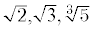and the like were not accepted as numbers during the highest period of Greek culture. Why not? Because the whole numbers and fractions had an obvious physical meaning whereas the irrational numbers did not. The only intuitive meaning that one could attach to irrationa1s was that they represented certain geometrical lengths, such as the diagonal of a square whose sides are 1. What then did the Greeks do? They rejected
irrationals as numbers and thought of them as lengths. In fact they converted all of algebra into geometry in order to work with lengths, areas and volumes that might otherwise have to be represented numerically by irrational numbers and they even solved quadratic equations geometrically.

The history of mathematics subsequent to the high period of Greek culture is quite the opposite of what one ordinarily conceives mathematics to be. The progress that was made in the use of irrational numbers is due to Alexandrian Greek civilization, which was a fusion of the classicai Greek, Egyptian and Babylonian civilizations, and to the Hindus and Arabs who were entirely empirically oriented. It was the Hindus who decided thatand their argument was that these irrationals could be "reckoned with like integers", that is, like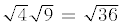Since the latter is obviously correct, as can be seen by taking the square root of each number, then so is.

Irrationa1 numbers were gradually accepted because of their utility and because familiarity breeds uncriticalness.

Negative numbers, introduced by the practical-minded about A.D. 600, did not gain acceptance for a thousand years. The reason: they lacked intuitive support. Some of the greatest mathematicians, Cardan, Vieta,Descartes, and Fermat, refused to work with negative numbers. The history of complex numbers is somewhat sinilar though they did not appear until about A.D. 1540 and only about two hundred years were required for these numbers to be used somewhat freely. A remark of the supreme mathematician Carl Friedrich Gauss is very pertinent. As is well known, he was one of the men who discovered the geometrica1 representation of complex nunmbers, and about this he said in 1831,

"Here [in this representation] the demonstration of an intuitive meaning of \/-1 is completely grounded and more is not needed in order to admit these quantities into the domain of the objects of arithmetic". Neither Descartes, Fermat, Newton, Leibniz, Euler, Lagrange, Gauss, or Cauchy could have given a definition of negative numbers or complex numbers, or irrationals for that matter. Yet all of them managed to work with these numbers quite satisfactorily, at least so far as their times employed these numbers. The history of the entire number system is pertinent not only because it shows how it was developed but also because algebra and analysis (the ca1cu1us and higher branches built on it) obviously utilize the number system, and whatever basis there was for the number system had to serve as the basis for a1gebra and analysis.

In the realm of algebra history has another significant tale to tell. The use of a letter to represent a fixed but unknown number dates from Greek times. However, the use of a letter or letters to stand for a whole class of numbers was not conceived of until the late sixteenth century. At that time François Vieta introduced expressions such as ax + b where a and b can be any (real) number..* The great merit of such genera1 expressions is that whatever we can prove about them is correct for aIl values of a, b, and x. Thus if we learn to solve the quadratic equation ax2 + bx + c = 0, we can solve all quadratic equations, because a, b, and c can stand for any numbers. The use of letters to stand for any numbers or even a restricted class of numbers is certainly, then, an enormous contribution and a seemingly simple one once it is pointed out. Yet during all the centuries that the Babylonians, Egyptians, Alexandrian Greeks, Hindus, and Arabs worked in algebra the idea of using letters for a class of numbers did not occur. These peoples did their a1gebra by working with concrete expressions such as 3x2 + 5x + 6 = 0. That is, they a1ways used numerical coefflciènts, and in fact most did not even use a symbol such as x for the unknown. They used words.

Why was the use of letters for genera1 coefficients so long delayed? The answer would seem to be that this device constitutes a higher level of abstraction in mathematics, a level farther removed from intuition. It is more difficult to think about ax2 + bx + c = 0 than about
3x2 + 5x + 6 = 0. Yet to reason deductively about the algebraic procedures for significant general expressions became possible only after these genera1 coefficients were introduced.

The history of the calculus is equally instructive. We shall not enter into the details of the concepts that lie at the foundation of that subject. But it may be sufficient to point out a few facts about its development. The great names in the creation of the calculus are, of course, Isaac Newton and Gottfried Wilhehn Leibniz. However, preceding them, Descartes, Fermat, Cavalieri, Pascai, Roberval, Barrow, and at least a dozen other well-known men made significant contributions. Despite the fact that so much was done before they did their work, neither Newton nor Leibniz could formulate correctly the basic concepts of the calculus. Newton wrote three major papers on the calculus and he put forth three editions of his masterpiece, The Mathematical Principles of Natural Philosophy. In each of these he gave a different explanation of the basic concept now called the derivative. Not one of these would be accepted from a beginning students of the calculus today. Leibniz in many papers was equally - unsuccessful. His first paper was described by the famous
Bernoulli brothers, James and John, as "
an enigma rather than an explication".

There were many attacks on the work of both Newton and Leibniz. Newton did not respond but Leibniz did. He objected to "overprecise critics" and he argued that we should not be led by excessive scrupulousness to reject the fruits of invention. However, the defects were there and the attacks continued throughout the eighteenth century. The difflculties in clarifying the basic concepts of the calculus were so great that the famous eighteenth-century mathematician Jean LeRond d'Alembert had to advise students, "Persist and faith will come to you".

In view of the vague, unclear and even incorrect foundations of the calculus one might expect that the subject would collapse. But before the adequate deductive structure was created not only had the ca1culus been extended and successfully applied but the vast subjects of ordinary and partial differential equations, the calculus of variations, differential geometry, and the theory of functions of a complex variable had been erected on the calcu1us. How did mathematicians achieve these tremendous creations? Clearly they thought intuitively. Physical argu-ments, pictures, generalizations based on simple known cases, and experience with mathematics all helped them to draw correct conclusions.

It is highly significant that the logical foundations of the number system, algebra, and analysis (the calculus and its extensions) were not erected until the last part of the nineteenth century. In other words, during the centuries in which the major branches of mathematics were built up there was no Iogical development for most of it. Apparently the intuitions of great men are more powerful than their logic.

What can we infer from this history? It seems clear that the concepts which have the most intuitive meaning, the whole numbers, fractions, and geometrical concepts, were accepted and utilized first. The less intuitive ones, irrational numbers, negative numbers, complex numbers, the use of letters for general coefficients, and concepts of the calculus, required many centuries either to be created or to be accepted. Moreover, when they were accepted, it was not the logic that induced mathematicians to adopt them but arguments by analogy, the physical meaning of some concepts, and the obtainment of correct scientific results. In other words, it was intuitive evidence that induced mathematicians to accept them. The logic always came long after the creations and evidently was harder to come by. Thus the history of mathematics suggests, though it does not prove, that the logical approach is more difficult.

There are many who would base another argument against the deductive approach on the evidence of history. The argument is essentially that each person must go through about the same experiences that his
forefathers did if he is to attain the level of thought that many generations have achieved. This argument has been advanced by many great mathematicians who have concerned themselves with pedagogy. Henri Poincaré, one of the greatest mathematicians of recent times, said in his
Foundations of Science (p. 437),

"The zoologists maintain that in a brief period the development of the embryo of an animal recapitulates the history of its ancestors of aIl geological epochs. It appears that it is the same in the development of the mind. The task of the educator is to make the mind of a child go through what his fathers have experienced, to pass rapidly through certain stages but not to omit any. For this purpose, the history of the science ought to be our guide".

The same thought has been expressed by one of the great mathematicians and teachers of the late nineteenth and early twentieth centuries. Felix Klein, in his Elementary Mathematics from an Advanced Standpoint (Dover reprint, 1945, Vol. 1, p. 268), says,

" In concluding thls discussion of the theory of assemblages [setsl we must again put the question which accompanies all of our lectures: How much of this can one use in the schools? From the standpoint of mathematical pedagogy, we must of course protest against putting such abstract and difficult things before the pupils too early. In order to give precise expression to my own view on this point, I should like to bring forward the biogenetic fundamental law, according to which the individual in his development goes through, in an abridged series, all the stages in the development of the species. Such thoughts have become today part and parcel of the general culture of everybody. Now, I think that instruction in mathematics, as well as in everything else, shou1d follow this law, at least in generai. Taking into account the native ability of youth, instruction should guide it slowly to higher ideas, and finally to abstract formulations, and in doing this it should follow the same road along which the human race has striven from its naive originai state to higher forms of knowledge. It is necessary to formulate this principle frequently, for there are always people who, after the fashion of the medieval scholastics, begin their instruction with the most general ideas, defending this method as the only scientific one. And yet this justification is based on anything but truth. To instruct scientifically can only mean to induce the person to think scientifically, but by no means to confront hlm, from the beginning, with cold scientiflcally polished systematics".

"An essential obstacle to the spreading of such a natural and truly scientific method of instruction is the lack of historical knowledge wbich so often makes itself felt. In order to combat this, I have made a point of introducing historical remarks into my presentation. By doing this I trust I have: made it clear to you how slowly all mathematical ideas have come into being, how they have nearly always appeared first in rather precursory form, and only after long development have crystallized into the deflnitive form so familiar in systematic presentation.

There is not much doubt that the difficulties the great mathematicians encounter are precisely the stumbling blocks that students experience and that no attempt to smother these difficulties with logical verbiage can succeed. If it took mathematicians a thousand years from the time first-class mathematics appeared to arrive at the concept of negative numbers - and it did - and if it took another thousand years for mathematicians to accept negative numbers - as it did - we may be sure that students will have difficulties with negative numbers. Moreover, the students will have to master these difficulties in about the same way that the mathematicians did, by gradually accustoming themselves to the new concepts, by working with them and by taking advantage of all the intuitive support that the teacher can muster.

One could of course argue that the growth of mathematics may indeed have proceeded as just described, but now that we have the proper logical structures for the number system, the calculus and other branches, we need not ask the students to repeat the fumblings of the masters. We can give students the correct approaches and they will understand them. This argument can be countered by the fact that the greatest mathematicians did try to build the logical foundations for the various subjects buf failed for centuries to do so. Their failure should serve as some evidence that the logical approaches are not easy to grasp. One can compress history and avoid many of the wasted efforts and pitfalls, but one caunot eliminate it. Of course, our students may be superior to the best mathematicians of the past.

But we shall not insist on the evidence of history. There are other weighty arguments against a purely deductive approach to elementary mathematics.

We might note, first of all, that we are not without experience in presenting mathematics deductively. Euclidean geometry has been presented in this manner for several centuries. Moreover, the intuitive meaning of this geometry is also evident to the student. Yet students have not been more successful in mastering geometry than algebra; nor have they left the geometry course with a feeling of elation because they have finally understood a branch of mathematics. If, then, the evidence of his-tory is not convincing, there must be other pedagogical arguments against a logical approach. They are not hard to find.

By the middle of the nineteenth century the various types of numbers and their properties were established on the basis of the uses made of them. Likewise, the properties of functions, derivatives and integrals used in the calcu1us were accepted on the basis of what seemed evident for the simplest functions or on the basis of the physical truth of the results obtained. The mathematicians then set about constructing logical foundations for the properties they had employed. In fact, the logic had to justify those properties, rather than determine them. Hence a very artificial and complicated structure of axioms and theorems was erected. The purpose of this structure was to satisfy the needs of professiona1 mathematicians who insist on deductive structure, but it was never intended as a pedagogical approach. Yet it is these logical foundations that the new mathematics employs to cultivate understanding.

The fact that utility determines the logical approach rather than the other way around is so basic in mathematics that the point warrants emphasis. Let us consider an example. To use addition of fractions in most rea1 situations, say, to add 1/2 ,and 1/3, we change both to sixths and then add 3/6 and 2/6 to obtain 5/6. However, when we multiply fractions we mu1tiply the numerators and multiply the denominators so that
1/2 x 1/3 = 1/6. We could "add" fractions by adding the numerators and adding the denominators and obtain 1/2 + 1/3 = 2/5. Why don't we use this latter inethod? It is simpler. But it does not fit experience. Having adopted a useful definition of addition the logical properties of addition must follow from the definition.

As another example we could consider matrix multiplication. It so happens that the uses to which matrices are put requires that the multiplication be noncommutative, though we could define a multiplication which is commutative. Since the multiplication must be noncommutative, the logical foundations of the theory of matrices must be suited to this fact. Therefore, logic does not dictate the contents of mathematics; the uses determine the logical structure. The logical organization is an afterthought and in a real sense is gilt on the lily.
[See Chapter 8, for the definition of a matrix]

In fact, if a student is really bright and he is told to cite the commutative axiom to justify, say, 3 X 4 = 4 x 3, he may very well ask, Why is the commutative axiom correct? The true answer is, of course, that we accept the commutative axiom because our experience with groups of objects tells us that 3 x 4 = 4 x 3. In other words, the commutative axiom is correct because 4 x 3 = 3 x 4 and not the other way around. The nor-mal student will parrot the words "commutative axiom" and he will, as Pascal put it in his Provincial Letters, "fix this term in his memory because it means nothing to his intelligence".

To repeat, the uses to which mathematics is put must be known to construct the logical foundations. Poincaré noted that in building up the number system from the positive whole numbers there are many different constructions one can make. Why do we take one rather than another?

"The choice is guided by the recollection of the intuitive notion in which this construction took place; without this recollection, the choice appears unjustified. But to understand a theory it is not sufficient to show that the path that one follows does not present obstacles; it is necessary to take account of the reasons that one chooses that path. Can one ever understand a theory if one builds it up right from the start in the definitive form that rigorous logic imposes, without some indications of the attempts which led to it? No; one does not really understand it; one cannot even retain it or one retains it only by learning it by heart".

Poincarés point may be made by considering the game of chess. To play the game it is not sufficient to know the rules for moving the pieces. With just this much knowledge one can see that a correct move has been made but one will not understand why one move has been made rather than another. The inner reason or strategy is not apparent. In the case of mathematics the inner reason is usage.

Actually the logical approach is misleading. In extending the number system from the natural numbers to the various other types, the new curriculum insists that the commutative and associative properties of the operations be retained. Why do we insist on these properties? The teachers know that the uses of the numbers call for these properties, but the students get the impression that these are necessary properties of all mathematical quantities. Why, then, do we not extend the commutative property to the multiplication of matrices? The answer is that the uses to which matrices are put require a non commutative multiplication. The logical approach gives the student an entirely false ïmpression of how mathematics develops.

Most proofs presented to students are also artificial for additiona1 reasons. When a mathematician seeks to prove a theorem he suspects is correct, he uses any means, however clumsy, indirect or devious, but perhaps more natural to the creative process, to make the proof. Once the theorem is proven he or his successors, now able to see how the essential difficulty was overcome, can usually devise a smoother or more direct proof. Some theorems have been reproven several times, each successive proof remodeling and simplifying the previous one and often including generalizations or stronger results. Thus, the final theorem and proof are far from the original natural thoughts. One should expect, then, that the student facing such a reworked, polished, and possibly more complicated resu1t would not be able to grasp it. Beyond attempting to refashion a proof to secure brevity and perhaps elegance, matbematicians like to erect logica1 structures in which many theorems follow from a small number of axioms. Fitting a theorem into such a structure may call for further juggling of the proof and cause still more difficulty to the student who seeks to understand it.

Many teachers, having delivered a series of such theorems and proofs, walk out of their classrooms very satisfied with themselves. But the students are not satisfied. They have not understood what was going on and all they can do is memorize what they heard. They were not involved in the origina1 thinking and derived no insight from the polished proofs. The deductive approach has been likened to the action of a fox who effaces his footsteps with his tail.

The insistence on a logical approach also deceives the student in another way. He is led to believe that mathematics is created by geniuses who start with axioms and reason directly from the axioms to the theorems. The student, unable to function in this manner, feels humbled and baffled but the obliging teacher is fully prepared to demonstrate genius in action Perhaps most of us do not need to be told how mathematics is created, but it may help to listen to the words of Felix Klein.

"You can often hear from non-mathematicians, especially from philosophers, that mathematics consists exclusively in drawing conclusions from clearly stated premises; and that in this process, it makes no difference what these premises signify, whether they are true or fa1se, provided only that they do not contradict one another. But a per. son who has done productive mathematical work will talk qulte differently. In fact these people [the non-mathematicians] are thinking only of the crystallized form into which finished mathematica1 theories are flnally cast. However, the investigator himself, in mathematics as in every other science, does not work in this rigorous deductive fashion. On the contrary, he makes essential use of his imagination and proceeds inductively aided by heuristic expedients. One can give numerous examples of mathematicians who have discovered theorems of the greatest importance which they were unable to prove. Should one then refuse to recognize this as a great accomplishment and in deference to the above deflnition insist that this is not mathematics? After all it is an arbitrary thing how the word is to be used, but no judgment of value can deny that the inductive work of the person who first announces the theorem is at least as valuable as the deductive work. of the one who proves it. For both are equally necessary and the tliscovery is the presupposition of the later conclusion".

It is intellectually dishonest to teach the deductive approach as though the results were acquired by logic.

The logical approach produces practica1 complications. If a student has to show, for example, that 4ab (ab + 3ac) = 4a2b2 + 12a2bc and if he has to justify each step, he will have to think carefully and give reasons for so many steps that he will take minutes to do what he should do almost automatically on the basis of experience with numbers. It is far preferable that the student should become so familiar with the basic properties such as distributivity, commutativity and associativity that he does not realize he is using them. -

Asking the students to cite axioms in the elementary operations with numbers is like asking an adult to justify each action he takes after getting up in the morning. Why should he bathe? Why should he brush his teeth? Why wear clothes? And so on. If a man should consciously consider and answer these questions he would never get to work. Most of what he does in the morning must be habitua1.

There is a story that a centipede was walking along leisurely when it met a toad. The toad remarked to the centipede, "Isn't it wonderful? You have one hundred feet and yet you know when to use each one". Thereupon the centipede began to think about which foot to use next and was unable to move.

Students should learn to become habitual about the elementary operations with numbers so that they do not have to think about them. After seeing that four sets of three blocks and three sets of four blocks both amount to twelve blocks, the students should accept the commutative principle as so obvious that it need not be mentioned. Moreover, instead of being obliged to consider this property for the natura1 numbers, the signed numbers, the rationa1 numbers, and so on, he should be induced to believe that all numbers possess these properties. In fact, we should do all we can to make the elementary operations so habitual that students do not have to think about them any more than one thinks when he ties his shoelaces. We should be grateful that stndents will accept unquestioningly facts that seem entirely reasonable to them whether on the basis of experience with numbers or intuitive arguments. If students do not see readily that 3 x a = a x 3, it is not because they lack familiarity with the commutative principle but rather because they fail to understand that a is just a number. When the time to teach a non commutative operation arrives, then the concept of commutativity can be discussed.

The need to make some of the work automatic was stressed by a man who certainly understood the role of deductive proof. The philosopher Alfred North Whitehead said in An Introduction to Mathematics,

"It is a profoundly erroneous truism, repeated by all copybooks, and by eminent people when they are making speeches, tbat we sbould cultivate the habit of thinging of what we are doing. The precise opposite is the case. Civilization advances by extending the number of important opera-tions which we can perform without thinlcing about them. Opertions of thought are like cavalry charges in a bat-tle - they are strictly limited in number, they require fresh horses, and must only be made at decisive, moments.

In many areas the present emphasis on the logical approach is sheer hypocrisy. What mathematician used the logical development of the complex number system to justify his operations with real or complex numbers? Yet this is what is taught to students as the way to learn the "truth" about numbers. How many mathematicians have ever satisfied themselves that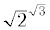is defined in the theory of irrational numbers or have examined the rigorous proof that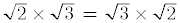? How many have ever worked through a rigorous development of Euclidean geometry, which would call for no reliance upon figures? Felix Klein did not hesitate to admit, "To follow a geometrical argumeut purely logically without having the figure on which the argument bears constantly before me is for me impossible".

As a matter of fact tbe attempt to be completely deductive ensnares the teacher in a trap. It is often necessary to include a proof which even the logic-oriented teachers concede to be too difficult for the student, such as the proof of the formula for the area of a circle in plane geometry. Many texts evade the issue by adopting as an axioin that the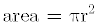where r is the radius. Surely if one can adopt axioms at will there is no need to prove anything. The only lesson the student will learn from such presentations is that if he is stuck he can adopt an axiom. Whereas in other matters students are asked to digest trivia thoroughly, the area axiom asks them to swallow a camel whole.

Moreover, by adopting axioms freely, numerous texts employ as many as seventy or eighty axioms. Since students are required to make proofs by citing axioms where these are the justifications for steps, the students are obliged to remember the seventy or eighty axioms. Tbis is an intolerable burden, an impossible one for students to carry. Yet such books claim to avoid memorization and to teach thinking and understanding. The mathe-matics teacher can no more afford to be profligate with axioms than to be parsimonious.

Other measures to avoid difficult proofs are equaliy harmful. In the presentation of the real number system the high school texts proceed axiomatically from the natural numbers. But when they get to the irrational numbers, whose logica1 development the authors recognize to be too difficult for the student, they resort to the number line. They show integers and fractions can be attached to points on the line and then note that some points do not have numbers assigned to them. The irrational numbers are then introduced as the numbers to be attached to these points. If the logical presentation of the rational numbers had any value it is dissipated by this meaningless introduction of the irrationals.

Every curriculum claims to teach the student how to discover results for himself. Discovery, contrasted with and opposed to the passive and uncritical acceptance of finished or polished statements of theorems and proofs, amounts to tbe creation or re-creation of mathematics by the student, possibly with the guidance of the teacher. How do the mathematicians create? Their first task is to divine a possible theorem. Having gotten this far they must do further creative work to find a proof. As the supreme mathematician Carl Friedrich Gauss put it, "I have got my result but I do not know yet how to get [prove] it". In the creative work imagination, intuition, experimentation, judicious guessing, trial and error, the use of analogies even of the vaguest sort, blundering and fumbling enter. Deductive proof plays little if any role.

Creativity presupposes flexibility in solving problems, and any ideas from any domain of mathematics should be entertained whether or not they fall within the confines of a particular axiomatic structure. The latter, in fact, acts as a straitjacket ou the mind.

What does logic contribute to the creation of concepts? What deductive process tells us to consider similar triangles or to consider the altitudes or the medians of a triangle? Logic alone is as incapable of leading to new ideas as grammar alone is incapable of leading to poetry and the theory of harmony to music. Creation has sometimes been described as the following process. The mathematician says A, writes B, means C, but D is what it should be. And D is in fact a splendid idea which emerges from tidying up the mess.

Some of the greatest ideas in matheniatics are not at all a matter of logic. Perhaps the best example is the realization that non-Euclidean geometry is applicable to physical space. The logical side, namely, pursuing the consequences of assuming a non-Euclidean parallel axiom, was a relatively simple task and was performed by Saccheri, Lambert, Legendre, Schweikart, Taurinus, and many others. But it was Gauss who first recognized that these new geometries are as applicable as Euclidean geometry. The consequences for mathematics were as revolutionary as the very creation of mathematics itself.

A foremost mathematician of our times, Henri Lebesgue, pointed out the subordinate role of logic.

"No discoveiy has been made in mathematics, or anywhere else for that matter, by an effort of deductive logic; it re-sults from the work of creative imagination which builds what seems to be truth, guided sometimes by analogies, sometimes by an esthetic ideal, but which does not hold at all on solid logical bases. Once a discovery is made, logic intervenes to act as a control; it is logic that ultimately decides whether the discovery is really true or is illusory; its role therefore, though considerable, is only secondary."

Thus the concentration on the deductive approach omits the vital work. It destroys the life and spirit of mathematics. The deductive formulation does dress up the real activity, but it conceals the flesh and blood. It is like the clothes which make the woman but are not thc woman. It is the last act in the development of a brauch of mathematics and, as one wise professor put it, when this is performed the subject is ready for burial. Logic may be a standard and an obligation of mathematics but it is not the essence any more than the grammatical structure is the essence of the Bible or Shakespeares works. The deductive structures which many mathematicians like to call mathematics are tbe dried-up stalks of the living plants. They are empty formalisms as opposed to real contents; they are shells of palaces.

Another argument advanced by the advocates of the new inathematics is that their emphasis on logical structure teaches students to think deductively. This is probably correct. But even if they do teach deductive logic, why is this so important? It is not the kind of thinking .that is useful in everyday life. The big problems and even the little ones that human beings are called upon to solve in life cannot be solved deductively. There are no self-evident axioms from which one can deduce what career to follow, whom to marry, or even whether to go to the movies. The real decisions call for judgment, and this is entirely difierent from deductive reasoning which leaves no room for judgment. The legal mind, the business nxind, and the political mind are much more relevant.

The modernists often espouse another argument for their approach. Mathematics can be enjoyed as a game played according to certain rules. But the purely axiomatic approach to mathematics must surely raise the question in inelligent students' minds, "Why shou1d I play this game according to these particular rules"?

In view of the many pedagogical shortcomings in the logical approach to mathematics it is not surprising that many perceptive mathematicians (there are some non-perceptive ones) have spoken out against the logical approach. Descartes deprecated logic in rather severe language.

"I found that, as for Logic, its syllogisnxs and the nxajority of its other precepts are useful rather in the communication of what we already know or . . . in speaking without judgment about things of which one is ignorant, than in the investigatiou of the unknown".

Roger Bacon said,

"Argument coucludes a question but it does not make us feel certain, or acqu1esce in the contemplation of a truth, except the truth also be found to be so by experience".

Pascal pointed out,

"Reason is the slow and tortuous method by which those who do not understand the truth discover it".

The logical approach is reminiscent of the reply Samuel Johnson gave to a man who persisted in asking for an explanation of his remarks. Johnson said, "I have found you an argument but I am not obliged to find you an understanding". The sterile, desiccated axiomatic approach has not promoted understanding. The formal logical style is one of the most devitalizing influences in the teaching of school mathematics. An ordered logical presentation of mathematics may have aesthetic appeal to the mathematician but serves as an anaesthetic to the student.

These arguments against an exclusively deductive approach to mathematics are not meant to imply tbat one should reject completely the use of deductive proof. It has a place which we shall discuss later (Chapter 11), but the most important fact about deductive proof is to keep it in its place.

Perhaps, after all there is some merit to the logical approach to mathematics. As Bertrand Russell put it in. his The Principles of Mathematics (p. 360), "It is one of the chief merits of proofs that they instill a certain scepticism as to the result proved". Henri Lebesgue pointed out another value of deductive proof: "Logic makes us reject certain arguments but it cannot make us believe any argument". One must respect but suspect mathematical proofs. Since one of the main objectives of mathematics education is to instill scepticism in the student, he is deriving at least one benefit from the current logical extravaganzas.

Professor Morris Kline

I am very grateful for the kind permission of Professor Kline's widow, Mrs Helen Kline for this book to be reproduced.

Version: 30th March 2018

 [HomePage] Prof Morris Kline